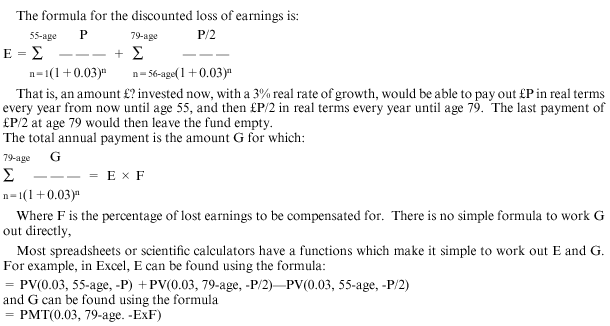Select Committee on Defence Minutes of Evidence

Annex E

CALCULATION OF THE GUARANTEED INCOME STREAM (GIS) IN THE DEFENCE ANALYTICAL SERVICE AGENCY SPREADSHEET MODEL

The "guaranteed income stream" is intended to compensate the individual for the loss of earnings due to the fact that ability to work has been impaired or lost. It is paid as a steady annual income uprated in line with inflation, comparable to an index linked annuity. Both the loss of earnings calculation and the conversion into an annual payment are based on the assumption that the individual dies aged 79.

The loss of earnings for the person is calculated as the person's current salary times the number of years until age 55. The assumed pension from age 55 (or from current age, if the award commences after age 55) until age 79 is calculated as half the current salary times the number of years. This gives a total for assumed "Loss of earnings".

However, because these lost earnings are in the future, their current value is less. If an amount of money were set aside now as an investment, a real rate of growth can be expected in that investment over time. For GIS purposes an indicative real rate has been assumed to be 3 per cent, as a long-term average. The future earnings are therefore deflated by 3 per cent per year to give the "present value" of the lost earnings.

The calculation for this is similar to a repayment mortgage calculation, with the repayment period until age 79 and assumed "interest rate" of 3 per cent. If a fund were set up with this discounted amount, which had a real rate of growth of 3 per cent per year, it would be just enough to pay out the lost earnings and would expire at the end of age 79. However, the GIS is paid and uprated until date of death regardless of age.

However, the individual will also be paid a service invaliding pension (SIP), and the compensation is reduced to allow for this. The reduction is based on the net present value of the projected SIP payments from now until age 79 (that is, the SIP payments are also discounted at a rate of 3 per cent per year to give the current "equivalent" of all future SIP payments). The reduced value (if any) of the GIS is then converted to an annual payment.

The formula used to derive this present value is set out in the appendix.

The percentage of lost earnings included in the compensation depends on the severity of disability, as expressed by the tariff. The percentages are:

 Tariffs 1-4: 100% Tariffs 5, 6: 75% Tariffs 7, 8: 50% Tariffs 9-11: 30% Tariffs 12-15: None

This assumes that less severely disabled people will still be able to find work after discharge from the Armed Forces.

FORMULAE USED IN CALCULATION OF GISThe actual GIS payment is the difference between G and the Service Invaliding Pension (SIP).

Example: An individual aged 23, current salary £20,000 on tariff 7.

Total present value of lost earnings = £473,542.29

This is just enough to pay £20,000 per year for the 32 years until age 55 and then £10,000 per year for the 24 years until age 79, assuming a 3 per cent real growth per year,

Compensation at 50 per cent for Tariff 7 = £236,771.15.

Annual payment, based on an initial fund of £236,771 growing at 3 per cent per year, paid out at a constant rate over 79-23 = 56 years = £8,780.53.

So that person would be entitled to a total payment each year of £8,781. If the SIP for that person's rank and reckonable length of service was, say £3,100, then they would get an annual top-up of £5,681 per year in addition to the SIP. This would be increased in line with inflation each year, and would be paid until their eventual death (whether this is before or after age 79).